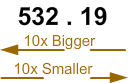# What is Decimal?

Print Rate 0 stars Common Core
Lesson size:
Message preview:
Someone you know has shared lesson with you:

To play this lesson, click on the link below:

https://www.turtlediary.com/lesson/what-is-decimal.html

Hope you have a good experience with this site and recommend to your friends too.

Login to rate activities and track progress.
Login to rate activities and track progress.

## Introduction

A decimal number is a number that has a decimal point.

A decimal point is a period that separates a decimal number into two parts. The number before the decimal point is called the whole part and the number after the decimal point is called the decimal part.

For example, in the number 532.19, 532 is the whole part and 19 is the decimal part.## Place Value

Just as "ones," "tens," "hundreds" and so on are used to describe place value for whole numbers, there are terms that describe place value for decimal numbers also.

The place before the decimal point is expressed as Ones, Tens, Hundreds, Thousands, and so on (from right to left on the left side of decimal point) and the place after the decimal point (from left to right after the decimal point) are expressed as Tenths, Hundredths, Thousandths, and so on.For example, the number 45.783 has 5 ones, 4 tens, 7 tenths, 8 hundredths and 3 thousandths.

NOTE: As we move to the left, each position is 10 times greater from ones, to tens, to hundreds, to thousands, and so on.

Tenths and hundredths are parts of 1. If you divide 1 into ten equal parts, each part is a tenth.

If you divide 1 into one hundred equal parts, each part is a hundredth, and so on.

So, as we move to the right of the decimal point, each position is 10 times less.While reading a decimal number, the digits on the left of the decimal point are read as whole number and the digits on the right of the decimal point are read as individual digits.

For example, 71.82 is read as seventy-one hundred point eight two or seventy-one hundred and eighty-two hundredths.

Note: Some decimals are smaller than one. These decimals start with a zero to show that there is nothing before the decimal point. For example: 0.28, 0.154.

Let's take a look at some examples.

### Example 1

What does the decimal number 532.19 mean?

Let us write the decimal number 532.19 in the place value table.

 Hundreds Tens Ones Tenths Hundredths 5 3 2 . 1 9

So, we have five hundreds, three tens, two ones, one tenth and nine hundredths.

This number is read as "Five hundred thirty two and nineteen hundredths", which means that the decimal number has 532 whole and 19 out of 100 parts of a whole.

### Example 2

What does the decimal number 0.24 mean?

Let us write the decimal number 0.24 in the place value table.

 Ones Tenths Hundredths 0 . 2 4

So, we have 2 tenths and 4 hundredths.

This number is read as "twenty four hundredths" which means 24 out of 100 parts of a whole.

## What is Decimal?

• A decimal number is a number that has a decimal point.
• A decimal point is a period that separates a decimal number into two parts. The number before the decimal point is called the whole part and the number after the decimal point is called the decimal part.
• The place before the decimal point is expressed as Ones, Tens, Hundreds, Thousands, and so on (from right to left on the left side of decimal point) and the place after the decimal point (from left to right after the decimal point) are expressed as Tenths, Hundredths, Thousandths, and so on.
• Tenths and hundredths are parts of 1. If you divide 1 into ten equal parts, each part is a tenth. If you divide 1 into one hundred equal parts, each part is a hundredth, and so on.
• While reading a decimal number, the digits on the left of the decimal point are read as whole number and the digits on the right of the decimal point are read as individual digits.
• Some decimals are smaller than one. These decimals start with a zero to show that there is nothing before the decimal point.

## Similar Lessons

Become premium member to get unlimited access.